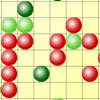#### You may also like### Ball Bearings

If a is the radius of the axle, b the radius of each ball-bearing, and c the radius of the hub, why does the number of ball bearings n determine the ratio c/a? Find a formula for c/a in terms of n.### Air Routes

Find the distance of the shortest air route at an altitude of 6000 metres between London and Cape Town given the latitudes and longitudes. A simple application of scalar products of vectors.### Epidemic Modelling

Use the computer to model an epidemic. Try out public health policies to control the spread of the epidemic, to minimise the number of sick days and deaths.

# Crystal Symmetry

##### Age 16 to 18 Challenge Level:

Caesium Chloride assumes a 'body centred cubic structure' in which each caesium ion is surrounded by $8$ chlorine ions located at the vertices of a cube, and vice-versa.

Mathematically, we can choose cartesian coordinates such that the ions lie on the integer lattice comprising the points $(l, m, n)$ with $l, m,n$ integers. The caesium ions are located at points with $l+m+n$ even and the chlorine ions at points with $l-m-n$ odd.

To start, make sure that you understand why the lattice points represent correctly a body centred cubic structure.

I can transform the points ${\bf v}$ in a lattice by multiplying by a constant matrix $M$ or adding a constant vector ${\bf c}$ through
$${\bf v} \rightarrow {\bf v} +{\bf c}\text{ or } {\bf v} \rightarrow M{\bf v}$$

Which of the following vectors and matrices preserve exactly the structure of the caesium chloride when they transform the lattice?

$$M =\pmatrix{1&0&0\cr 0&0&-1\cr 0&1&0}\,,\pmatrix{3&0&0\cr 0&1&0\cr 0&0&2}\,,\pmatrix{1&1&-1\cr -1&1&1\cr 1&-1&1}$$

$${\bf c} = \pmatrix{1\cr 0\cr 0}\,,\pmatrix{1\cr 1\cr 0}\,,\pmatrix{2\cr 2\cr 2}\,,\pmatrix{4\cr -2\cr -8}$$

In each case prove why the crystal structure is preserved, or explain what goes wrong.

Using your geometrical intuition as a guide, investigate the types of transformations which will leave the crystal invariant.